## ML Aggarwal Class 10 Solutions for ICSE Maths Chapter 3 Shares and Dividends Chapter Test

These Solutions are part of ML Aggarwal Class 10 Solutions for ICSE Maths. Here we have given ML Aggarwal Class 10 Solutions for ICSE Maths Chapter 3 Shares and Dividends Chapter Test

More Exercises

Question 1.
If a man received ₹1080 as dividend from 9% ₹20 shares, find the number of shares purchased by him.
Solution:
Income on one share = $$\\ \frac { 9 }{ 100 }$$ x 20
= Rs $$\\ \frac { 9 }{ 5 }$$
.’. No. of shares = 1080 x $$\\ \frac { 5 }{ 9 }$$
= 120 x 5 = 600 Ans.

Question 2.
Find the percentage interest on capital invested in 18% shares when a Rs 10 share costs Rs 12.
Solution:
Dividend on one share = 18% of Rs 10
= $$\\ \frac { 18\times 10 }{ 100 }$$
= Rs $$\\ \frac { 9 }{ 5 }$$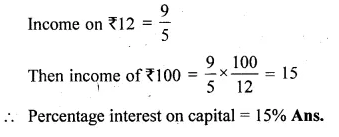Question 3.
Rohit Kulkami invests Rs 10000 in 10% Rs 100 shares of a company. If his annual dividend is Rs 800, find :
(i) The market value of each share.
(ii) The rate percent which he earns on his investment.
Solution:
Investment = Rs 10000
Face value of each share = Rs 100
Rate of dividend = 10%
Annual dividend = Rs 800Question 4.
At what price should a 9% Rs 100 share be quoted when the money is worth 6% ?
Solution:
If interest is 6 then investment = Rs 100
and if interest is 9, then investment
= Rs $$\\ \frac { 100\times 9 }{ 6 }$$
= Rs 150
Market value of each share = Rs 150 Ans

Question 5.
By selling at Rs 92, some 2.5% Rs 100 shares and investing the proceeds in 5% Rs 100 shares at Rs 115, a person increased his annual income by Rs 90. Find:
(i) the number of shares sold.
(ii) the number of shares purchased.
(iii) the new income.
(iv) the rate percent which he earns on his investment.
Solution:
Rate of dividend = 2.5% and market price = Rs 92
Let number of shares purchased = x.
Selling price of x shares = 92 x
Income from investing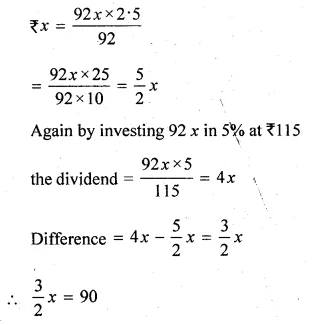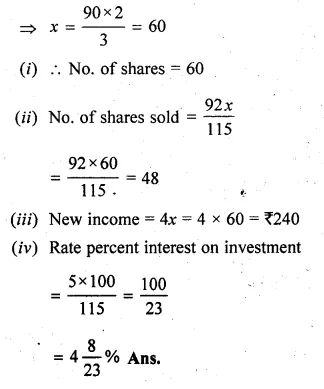Question 6.
A man has some shares of Rs. 100 par value paying 6% dividend. He sells half of these at a discount of 10% and invests the proceeds in 7% Rs. 50 shares at a premium of Rs. 10. This transaction decreases his income from dividends by Rs. 120. Calculate:
(i) the number of shares before the transaction.
(ii) the number of shares he sold.
(iii) his initial annual income from shares.
Solution:
Let no. of shares = x
Value of x shares = x × 100 = 100 x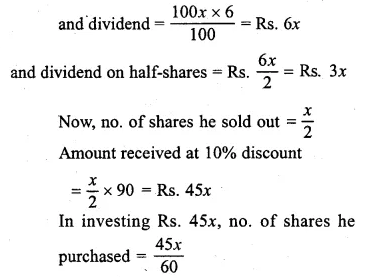Question 7.
Divide Rs. 101520 into two parts such that if one part is invested in 8% Rs. 100 shares at 8% discount and the other in 9% Rs. 50 shares at 8% premium, the annual incomes are equal.
Solution:
Total investment = Rs. 101520
Let investment in first part = x
and in second part = (101520 – x)
Market value of first kind of shares = Rs. 100 – Rs. 8
= Rs. 92
and rate of dividend = 8%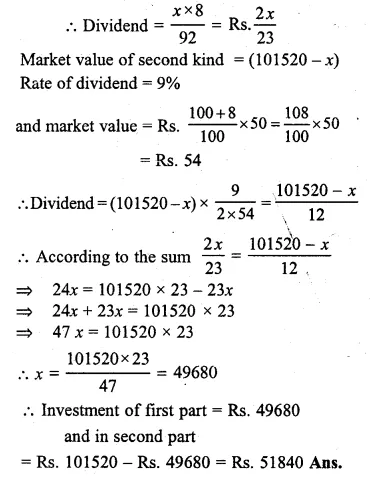Question 8.
A man buys Rs. 40 shares of a company which pays 10% dividend. He buys the shares at such a price that his profit is 16% on his investment. At what price did he buy each share ?
Solution:
Face value of each share = Rs. 40
Dividend = 10%
Gain on investment = 10%Hope given ML Aggarwal Class 10 Solutions for ICSE Maths Chapter 3 Shares and Dividends Chapter Test are helpful to complete your math homework.

If you have any doubts, please comment below. Learn Insta try to provide online math tutoring for you.